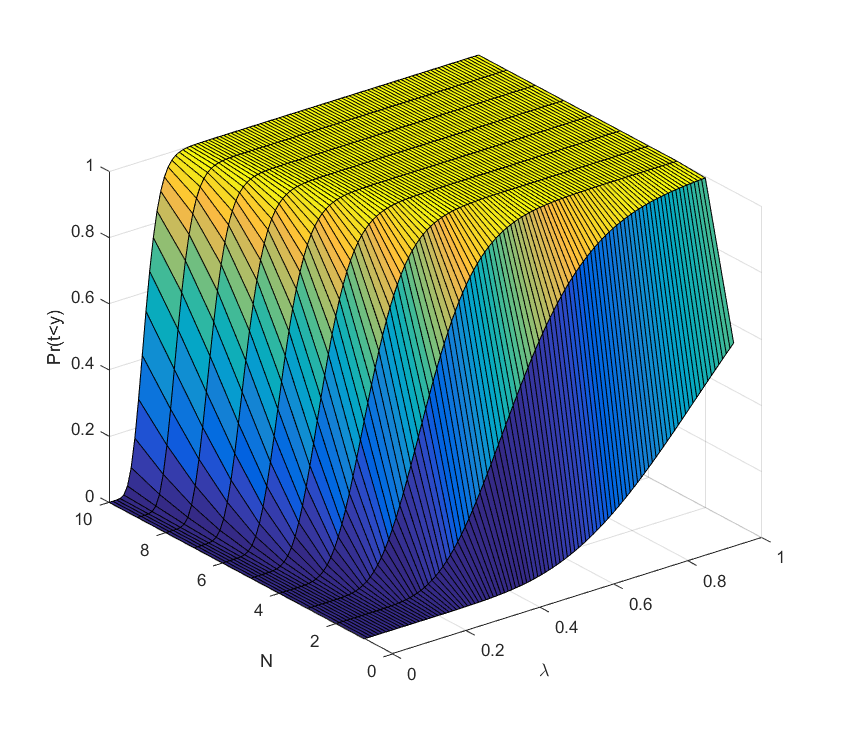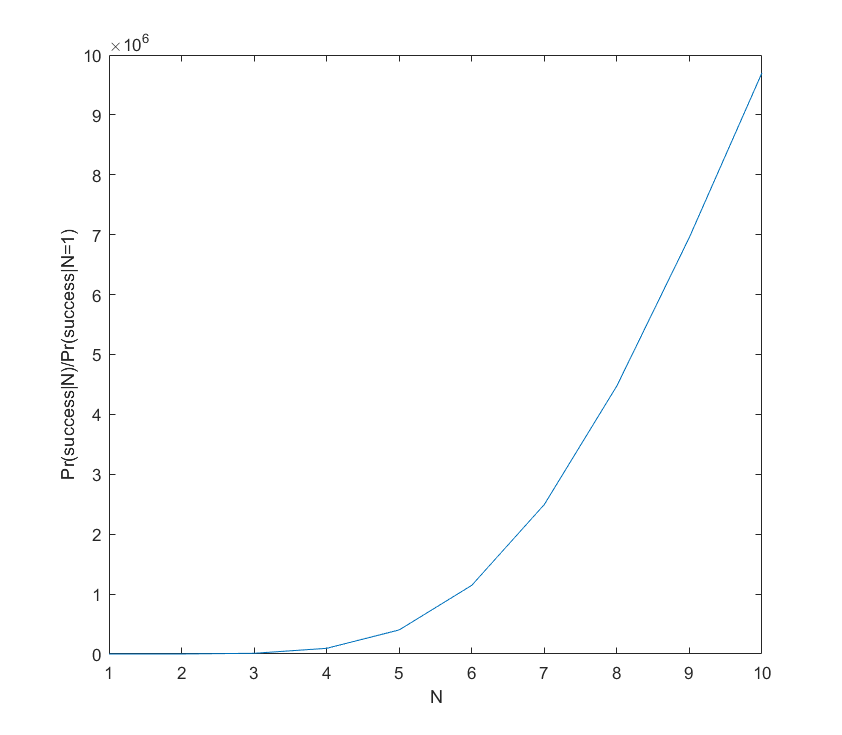# Adding cooks to the broth

If there are$k$ key ideas needed to produce some important goal (like AI), there is a constant probability per researcher-year to come up with an idea, and the researcher works for$y$ years, what is the the probability of success? And how does it change if we add more researchers to the team?

The most obvious approach is to think of this as y Bernouilli trials with probability p of success, quickly concluding that the number of successes n at the end of y years will be distributed as$\mathrm{Pr}(n)=\binom{y}{n}p^n(1-p)^{y-n}$. Unfortunately, then the actual answer to the question will be$\mathrm{Pr}(n\geq k) = \sum_{n=k}^y \binom{y}{n}p^n(1-p)^{y-n}$ which is a real mess…

A somewhat cleaner way of thinking of the problem is to go into continuous time, treating it as a homogeneous Poisson process. There is a rate$\lambda$ of good ideas arriving to a researcher, but they can happen at any time. The time between two ideas will be exponentially distributed with parameter$\lambda$. So the time$t$ until a researcher has$k$ ideas will be the sum of$k$ exponentials, which is a random variable distributed as the Erlang distribution:$f(t; k,\lambda)=\lambda^k t^{k-1} e^{-\lambda t} / (k-1)!$.

Just like for the discrete case one can make a crude argument that we are likely to succeed if$y$ is bigger than the mean$k/\lambda$ (or$k/p$) we will have a good chance of reaching the goal. Unfortunately the variance scales as$k/\lambda^2$ – if the problems are hard, there is a significant risk of being unlucky for a long time. We have to consider the entire distribution.

Unfortunately the cumulative density function in this case is$\mathrm{Pr}(t which is again not very nice for algebraic manipulation. Still, we can plot it easily.

Before we do that, let us add extra researchers. If there are$N$ researchers, equally good, contributing to the idea generation, what is the new rate of ideas per year? Since we have assumed independence and a Poisson process, it just multiplies the rate by a factor of$N$. So we replace$\lambda$ with$\lambda N$ everywhere and get the desired answer.This is a plot of the case$k=10, y=10$.

What we see is that for each number of scientists it is a sigmoid curve: if the discovery probability is too low, there is hardly any chance of success, when it becomes comparable to$k/N$ it rises, and sufficiently above we can be almost certain the project will succeed (the yellow plateau). Conversely, adding extra researchers has decreasing marginal returns when approaching the plateau: they make an already almost certain project even more certain. But they do have increasing marginal returns close to the dark blue “floor”: here the chances of success are small, but extra minds increase them a lot.

We can for example plot the ratio of success probability for$\lambda=0.09$ to the one researcher case as we add researchers:Even with 10 researchers the success probability is just 40%, but clearly the benefit of adding extra researchers is positive. The curve is not quite exponential; it slackens off and will eventually become a big sigmoid. But the overall lesson seems to hold: if the project is a longshot, adding extra brains makes it roughly exponentially more likely to succeed.

It is also worth recognizing that in this model time is on par with discovery rate and number of researchers: what matters is the product$\lambda y N$ and how it compares to$k$.

This all assumes that ideas arrive independently, and that there are no overheads for having a large team. In reality these things are far more complex. For example, sometimes you need to have idea 1 or 2 before idea 3 becomes possible: that makes the time$t_3$ of that idea distributed as an exponential plus the distribution of$\mathrm{min}(t_1,t_2)$. If the first two ideas are independent and exponential with rates$\lambda, \mu$, then the minimum is distributed as an exponential with rate$\lambda+\mu$. If they instead require each other, we get a non-exponential distribution (the pdf is$\lambda e^{-\lambda t} + \mu e^{-\mu t} - (\lambda+\mu)e^{-(\lambda+\mu)t}$). Some discoveries or bureaucratic scalings may change the rates. One can construct complex trees of intellectual pathways, unfortunately quickly making the distributions impossible to write out (but still easy to run Monte Carlo on). However, as long as the probabilities and the induced correlations small, I think we can linearise and keep the overall guess that extra minds are exponentially better.

In short: if the cooks are unlikely to succeed at making the broth, adding more is a good idea. If they already have a good chance, consider managing them better.